# Berger inequality

(diff) ← Older revision | Latest revision (diff) | Newer revision → (diff)

For a compact Riemannian manifold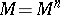, let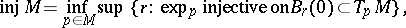whereis the ball around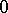with radius, be the injectivity radius, and set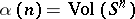. Then the inequalityholds, with equality if and only ifis isometric to the standard sphere with diameter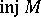.

This inequality relies on the Kazdan inequality applied to the Jacobi equation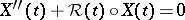for operatorson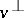for a unit vector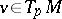. Here,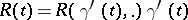is the curvature operator,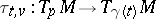is the parallel transport along the geodesic ray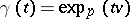, and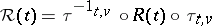is the parallel translated curvature operator on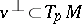.

How to Cite This Entry:
Berger inequality. Encyclopedia of Mathematics. URL: http://encyclopediaofmath.org/index.php?title=Berger_inequality&oldid=15922
This article was adapted from an original article by H. Kaul (originator), which appeared in Encyclopedia of Mathematics - ISBN 1402006098. See original article